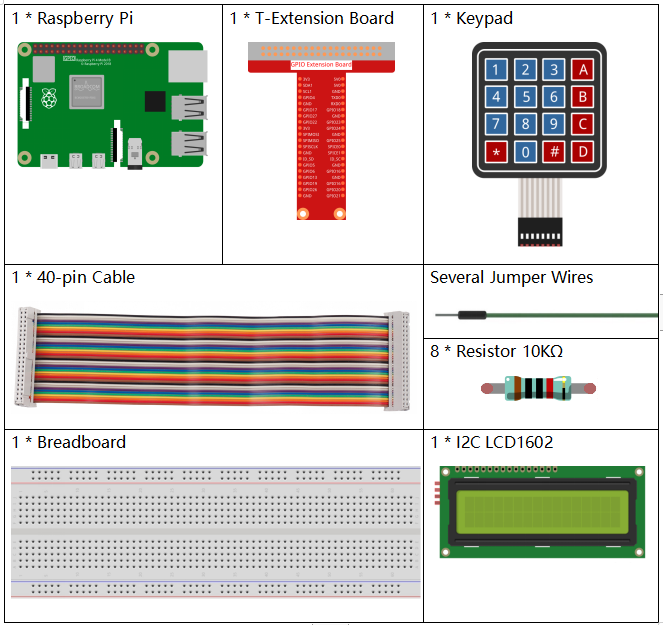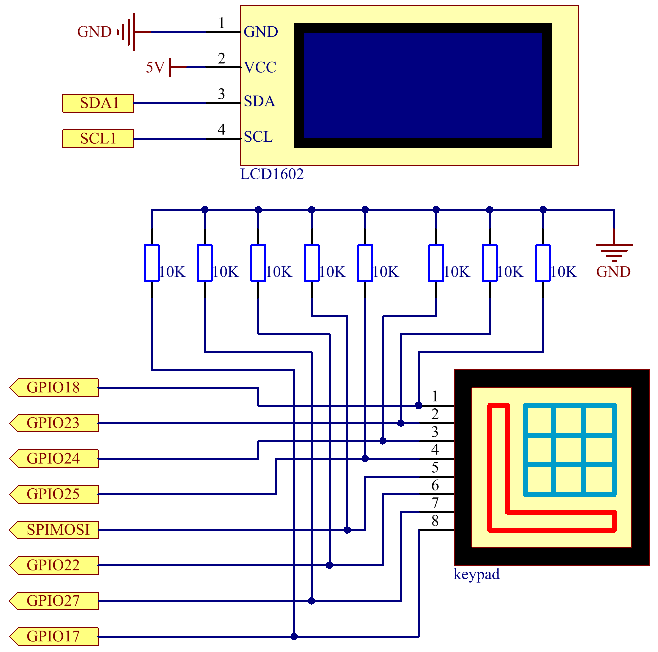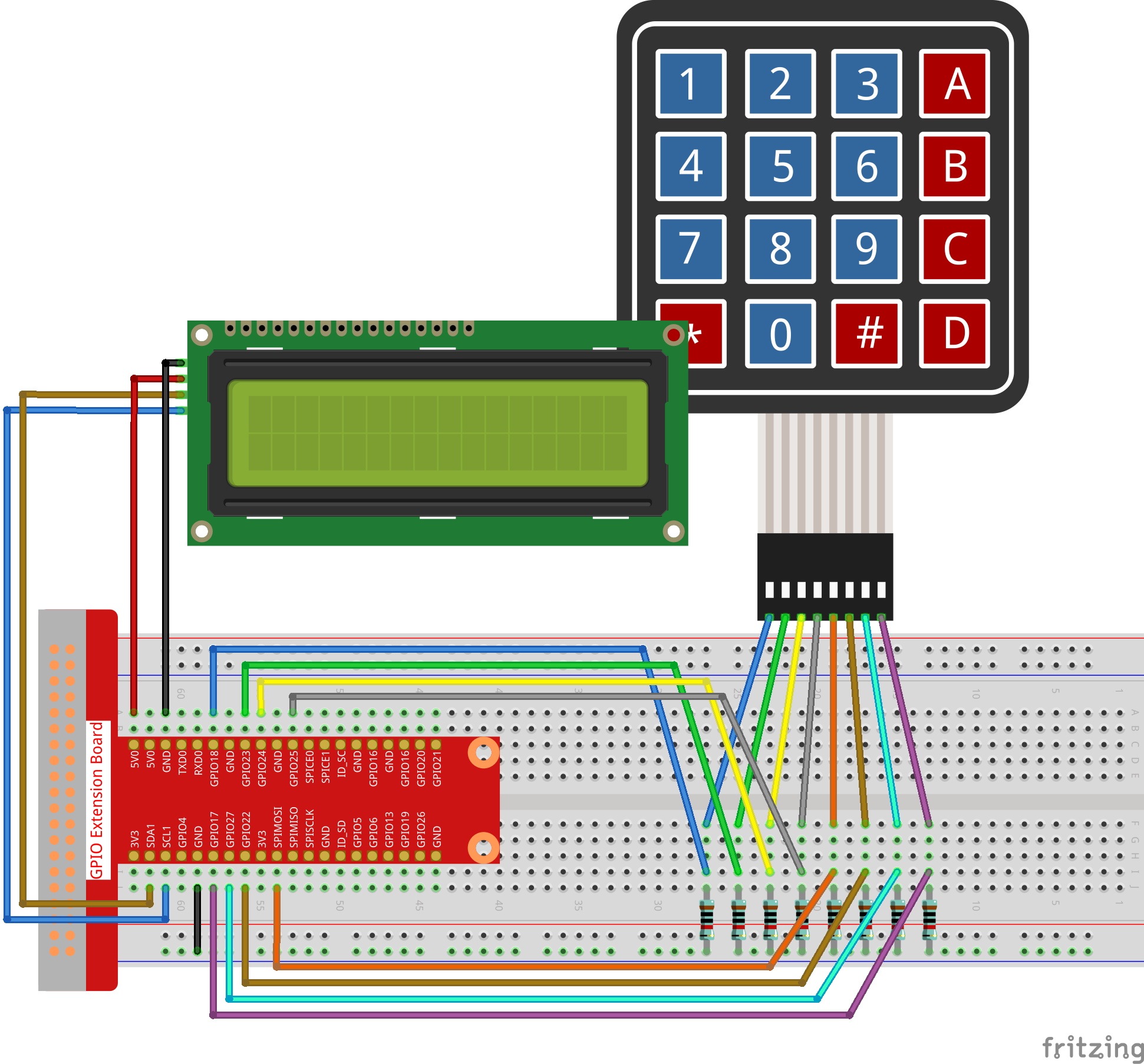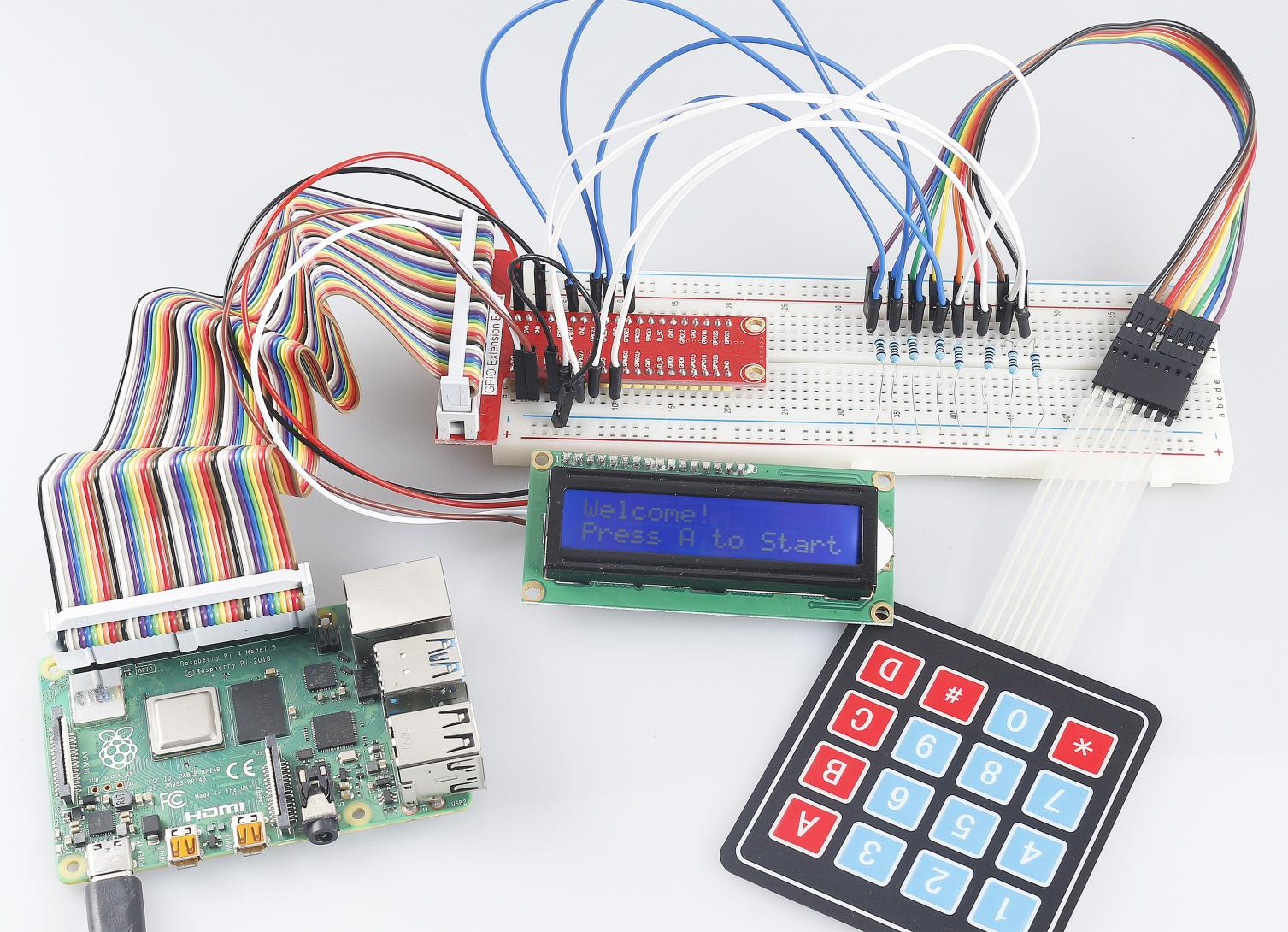# 4.1.17 GAME– Guess Number¶

## Introduction¶

Guessing Numbers is a fun party game where you and your friends take turns inputting a number (0~99). The range will be smaller with the inputting of the number till a player answers the riddle correctly. Then the player is defeated and punished. For example, if the lucky number is 51 which the players cannot see, and the player ① inputs 50, the prompt of number range changes to 50~99; if the player ② inputs 70, the range of number can be 50~70; if the player ③ inputs 51, this player is the unlucky one. Here, we use keypad to input numbers and use LCD to output outcomes.

## Components¶## Schematic Diagram¶

 T-Board Name physical wiringPi BCM GPIO18 Pin 12 1 18 GPIO23 Pin 16 4 23 GPIO24 Pin 18 5 24 GPIO25 Pin 22 6 25 SPIMOSI Pin 19 12 10 GPIO22 Pin 15 3 22 GPIO27 Pin 13 2 27 GPIO17 Pin 11 0 17 SDA1 Pin 3 SDA1(8) SDA1(2) SCL1 Pin 5 SCL1(9) SDA1(3)## Experimental Procedures¶

Step 1: Build the circuit.Step 2: Setup I2C (see I2C Configuration.)

Step 3: Change directory.

```cd /home/pi/raphael-kit/python/
```

Step 4: Run.

```sudo python3 4.1.17_GAME_GuessNumber.py
```

After the program runs, there displays the initial page on the LCD:

```Welcome!
Press A to go!
```

Press ‘A’, and the game will start and the game page will appear on the LCD.

```Enter number:
0 ‹point‹ 99
```

A random number ‘point’ is produced but not displayed on the LCD when the game starts, and what you need to do is to guess it. The number you have typed appears at the end of the first line till the final calculation is finished. (Press ‘D’ to start the comparation, and if the input number is larger than 10, the automatic comparation will start.)

The number range of ‘point’ is displayed on the second line. And you must type the number within the range. When you type a number, the range narrows; if you got the lucky number luckily or unluckily, there will appear “You’ve got it!”

Note

• If you get the error `FileNotFoundError: [Errno 2] No such file or directory: '/dev/i2c-1'`, you need to refer to I2C Configuration to enable the I2C.

• If you get `ModuleNotFoundError: No module named 'smbus2'` error, please run `sudo pip3 install smbus2`.

• If the error `OSError: [Errno 121] Remote I/O error` appears, it means the module is miswired or the module is broken.

Code

Note

You can Modify/Reset/Copy/Run/Stop the code below. But before that, you need to go to source code path like `raphael-kit/python`. After modifying the code, you can run it directly to see the effect.

```#!/usr/bin/env python3

import RPi.GPIO as GPIO
import time
import LCD1602
import random

##################### HERE IS THE KEYPAD LIBRARY TRANSPLANTED FROM Arduino ############
#class Key:Define some of the properties of Key

def __init__(self, rowsPins, colsPins, keys):
self.rowsPins = rowsPins
self.colsPins = colsPins
self.keys = keys
GPIO.setwarnings(False)
GPIO.setmode(GPIO.BCM)
GPIO.setup(self.rowsPins, GPIO.OUT, initial=GPIO.LOW)
GPIO.setup(self.colsPins, GPIO.IN, pull_up_down=GPIO.PUD_DOWN)

pressed_keys = []
for i, row in enumerate(self.rowsPins):
GPIO.output(row, GPIO.HIGH)
for j, col in enumerate(self.colsPins):
index = i * len(self.colsPins) + j
if (GPIO.input(col) == 1):
pressed_keys.append(self.keys[index])
GPIO.output(row, GPIO.LOW)
return pressed_keys

################ EXAMPLE CODE START HERE ################

count = 0
pointValue = 0
upper=99
lower=0

def setup():
rowsPins = [18,23,24,25]
colsPins = [10,22,27,17]
keys = ["1","2","3","A",
"4","5","6","B",
"7","8","9","C",
"*","0","#","D"]
last_key_pressed = []
LCD1602.init(0x27, 1)    # init(slave address, background light)
LCD1602.clear()
LCD1602.write(0, 0, 'Welcome!')
LCD1602.write(0, 1, 'Press A to Start!')

def init_new_value():
global pointValue,upper,count,lower
pointValue = random.randint(0,99)
upper = 99
lower = 0
count = 0
print('point is %d' %(pointValue))

def detect_point():
global count,upper,lower
if count > pointValue:
if count < upper:
upper = count
elif count < pointValue:
if count > lower:
lower = count
elif count == pointValue:
count = 0
return 1
count = 0
return 0

def lcd_show_input(result):
LCD1602.clear()
if result == 1:
LCD1602.write(0,1,'You have got it!')
time.sleep(5)
init_new_value()
lcd_show_input(0)
return
LCD1602.write(0,0,'Enter number:')
LCD1602.write(13,0,str(count))
LCD1602.write(0,1,str(lower))
LCD1602.write(3,1,' < Point < ')
LCD1602.write(13,1,str(upper))

def loop():
while(True):
result = 0
if len(pressed_keys) != 0 and last_key_pressed != pressed_keys:
if pressed_keys == ["A"]:
init_new_value()
lcd_show_input(0)
elif pressed_keys == ["D"]:
result = detect_point()
lcd_show_input(result)
elif pressed_keys in keys:
if pressed_keys in list(["A","B","C","D","#","*"]):
continue
count = count * 10
count += int(pressed_keys)
if count >= 10:
result = detect_point()
lcd_show_input(result)
print(pressed_keys)
last_key_pressed = pressed_keys
time.sleep(0.1)

# Define a destroy function for clean up everything after the script finished
def destroy():
# Release resource
GPIO.cleanup()
LCD1602.clear()

if __name__ == '__main__':     # Program start from here
try:
setup()
while True:
loop()
except KeyboardInterrupt:   # When 'Ctrl+C' is pressed, the program destroy() will be executed.
destroy()
```

Code Explanation

At the beginning part of the code are the functional functions of keypad and I2C LCD1602. You can learning more details about them in 1.1.7 I2C LCD1602 and 2.1.8 Keypad.

Here, what we need to know is as follows:

```def init_new_value():
global pointValue,upper,count,lower
pointValue = random.randint(0,99)
upper = 99
lower = 0
count = 0
print('point is %d' %(pointValue))
```

The function produces the random number ‘point’ and resets the range hint of the point.

```def detect_point():
global count,upper,lower
if count > pointValue:
if count < upper:
upper = count
elif count < pointValue:
if count > lower:
lower = count
elif count == pointValue:
count = 0
return 1
count = 0
return 0
```

`detect_point()` compares the input number (count) with the produced “point”. If the comparing outcome is that they are not same, count will assign values to upper and lower and return ‘0’; otherwise, if the outcome indicates they are same, there returns ‘1’.

```def lcd_show_input(result):
LCD1602.clear()
if result == 1:
LCD1602.write(0,1,'You have got it!')
time.sleep(5)
init_new_value()
lcd_show_input(0)
return
LCD1602.write(0,0,'Enter number:')
LCD1602.write(13,0,str(count))
LCD1602.write(0,1,str(lower))
LCD1602.write(3,1,' < Point < ')
LCD1602.write(13,1,str(upper))
```

This function works for displaying the game page.

`str(count)`: Because `write()` can only support the data type — string, `str()` is needed to convert the number into string.

```def loop():
while(True):
result = 0
if len(pressed_keys) != 0 and last_key_pressed != pressed_keys:
if pressed_keys == ["A"]:
init_new_value()
lcd_show_input(0)
elif pressed_keys == ["D"]:
result = detect_point()
lcd_show_input(result)
elif pressed_keys in keys:
if pressed_keys in list(["A","B","C","D","#","*"]):
continue
count = count * 10
count += int(pressed_keys)
if count >= 10:
result = detect_point()
lcd_show_input(result)
print(pressed_keys)
last_key_pressed = pressed_keys
time.sleep(0.1)
```

`main()` contains the whole process of the program, as show below:

1. Initialize I2C LCD1602 and Keypad.

2. Judge whether the button is pressed and get the button reading.

3. If the button ‘A’ is pressed, a random number 0-99 will appear then the game starts.

4. If the button ‘D’ is detected to have been pressed, the program will enter into the outcome judgement.

5. If the button 0-9 is pressed, the value of count will be changed; if the count is larger than 10, then the judgement starts.

6. The changes of the game and its values are displayed on LCD1602.

## Phenomenon Picture¶